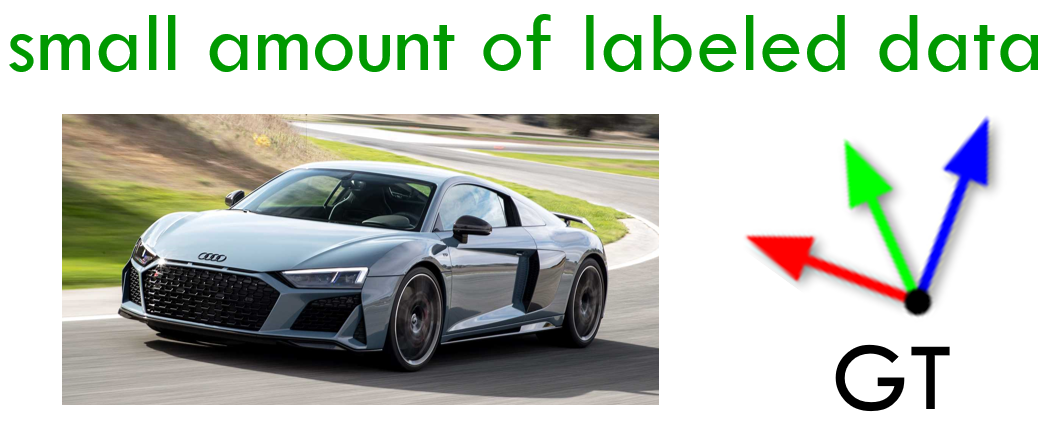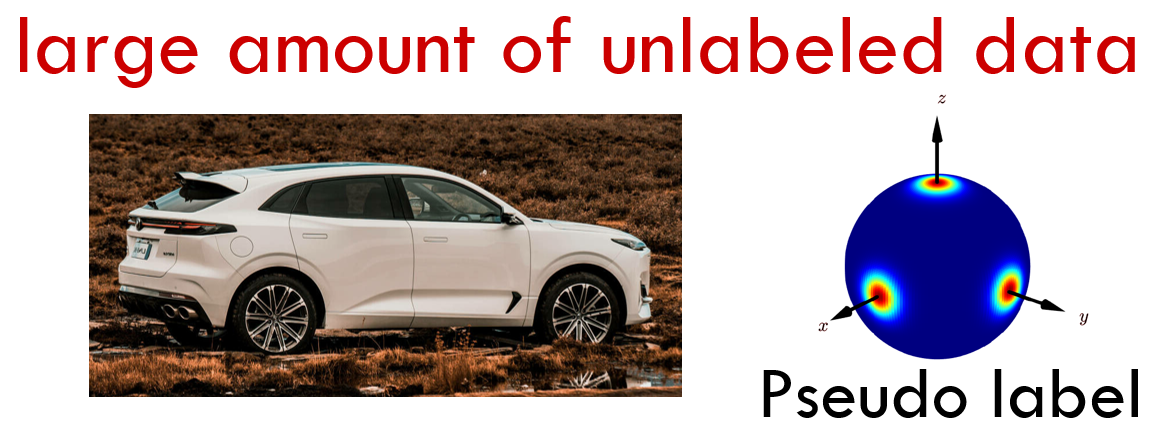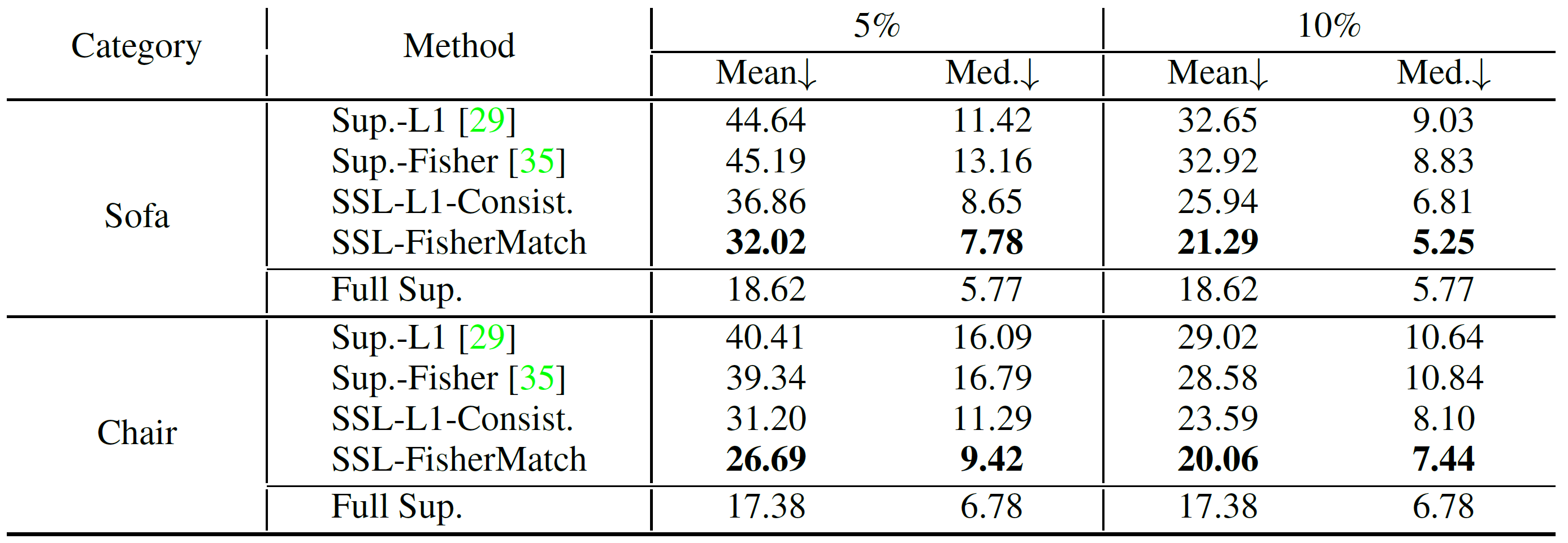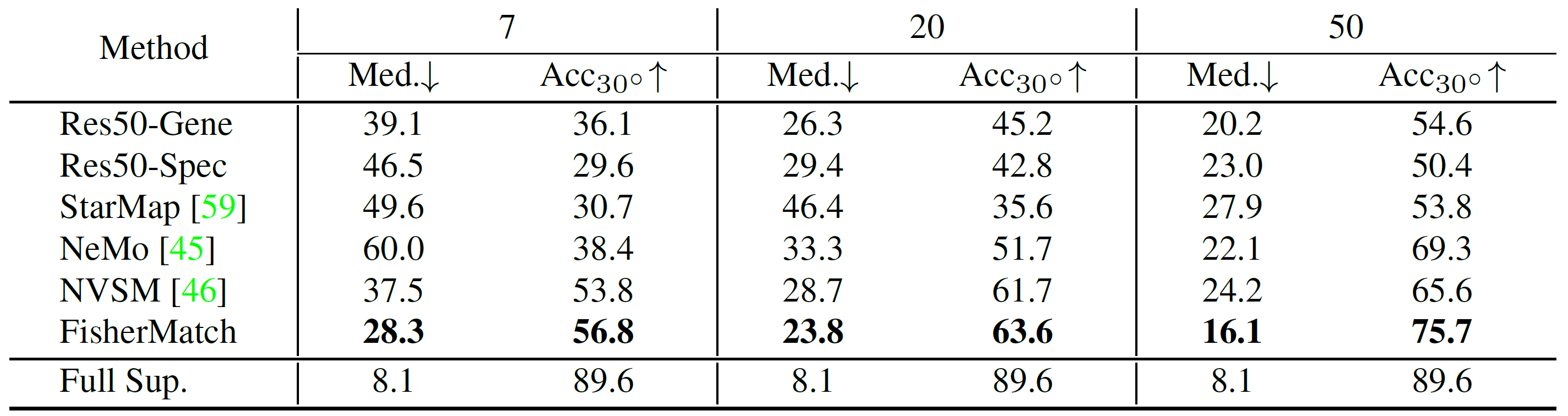# FisherMatch: Semi-Supervised Rotation Regression via Entropy-based Filtering

CVPR2022 (Oral Presentation)
Peking University

## Introduction

Estimating the 3DoF rotation from a single RGB image is an important yet challenging problem in computer vision, graphics and robotics. Though recent supervised rotation regression works achieve good performance, they rely on a large amount of labeled data, which can be expensive and time-consuming to obtain, thus becomes one of the obstacles to improving rotation regression.

To reduce the amount of supervision, semi-supervised learning (SSL) has been a powerful approach, where many progresses have witnessed in classification tasks recently. However, few works address semi-supervised regression, especially semi-supervised rotation regression. Given that rotation space SO(3) is a non-Euclidean manifold, a general regression algorithm needs to be tailored, taking the nonlinear structure of the rotation space into account, which makes semi-supervised rotation regression a more challenging and less studied topic.In this work, for the first time, we propose a general framework, namely FisherMatch, for semi-supervised rotation regression, without assuming any domain-specific knowledge or paired data. Inspired by the popular semi-supervised approach, FixMatch, we propose to leverage pseudo label filtering to facilitate the information flow from labeled data to unlabeled data in a teacher-student mutual learning framework. However, incorporating the pseudo label filtering mechanism into semi-supervised rotation regression is highly non-trivial, mainly due to the lack of a reliable confidence measure for rotation prediction.

We propose to leverage matrix Fisher distribution to build a probabilistic model of rotation and devise a matrix Fisher-based regressor for jointly predicting rotation along with its prediction uncertainty. We then propose to use the entropy of the predicted distribution as a confidence measure, which enables us to perform pseudo label filtering for rotation regression.

Our extensive experiments show that our method can work well even under very low labeled data ratios on different benchmarks, achieving significant and consistent performance improvement over supervised learning and other semi-supervised learning baselines.

## Method

### Probabilistic Modeling of Rotation

Matrix Fisher distribution is a probability distribution over SO(3) for rotation matrices, whose probability density function is in the form of

where parameter A is an arbitrary 3×3 matrix and F(A) is the normalizing constant. The mode and dispersion of the distribution can be computed from computing singular value decomposition of the parameter A.

Another important probabilistic model for rotation is Bingham distribution for unit quaternions. The probability density function is defined as

where M is a 4×4 orthogonal matrix and Z is a 4×4 diagonal matrix. The first column of parameter M indicates the mode and the remaining columns describe the orientation of dispersion while the corresponding entries describe the strength of the dispersion.

### Pipeline

Our matrix Fisher based-rotation regressor Φ takes an RGB image x as input and outputs the parameter A of the predicted matrix Fisher distribution. We leverage a teacher-student mutual learning framework composed of a learnable student model Φs and an exponential-moving-average (EMA) teacher model Φt. On labeled data, the student network is trained by the ground-truth labels with the supervised loss; while on unlabeled data, the student model takes the pseudo labels from the EMA teacher.

Inspired by FixMatch, we only want the accurate predictions from the teacher model to "teach" the student model. Otherwise, noisy pseudo labels may slow down the training procedure, or even do harm to the whole process. For depicting the confidence of a predicted distribution, we propose to use entropy, which is widely used in statistics acting as the degree of disorder or randomness in the system, as a measure of uncertainty. A lower entropy generally indicates a more peaked distribution which exhibits less uncertainty and higher confidence.

For pseudo label filtering, we set a fixed entropy threshold τ , and only reserve the prediction as a pseudo label if its entropy is lower than the threshold.

## Experiment

On common benchmark datasets of object rotation estimation from RGB images (ModelNet10-SO(3) and Pascal3D+) under various labeled data ratios, our experiment demonstrates a significant and consistent performance improvement over supervised learning and other semi-supervised learning baselines.Comparing our proposed FisherMatch with the baselines on ModelNet10-SO(3) under different ratios of labeled data.Comparing our proposed FisherMatch with the baselines on the 6 categories of Pascal3D+ dataset with few annotations (7, 20, 50 images).

Shown in the curves, as the SSL goes on, the improving model leads to more confident predictions indicated by the increasing pseudo label coverage, while the pseudo label quality still keeps stable, resulting in the steady performance increasing on both unlabeled dataset and test dataset.